Anúncio

# Decision Trees for Classification: A Machine Learning Algorithm

11 de Sep de 2018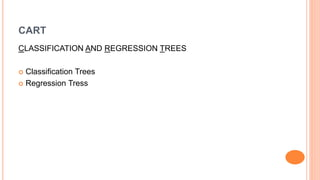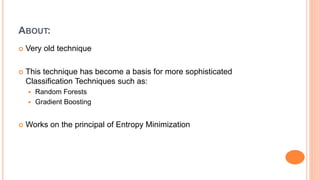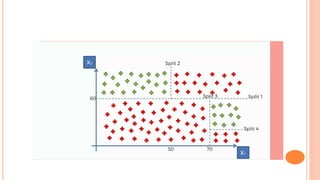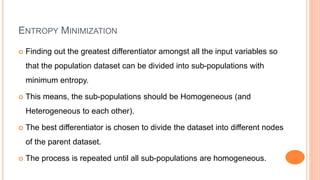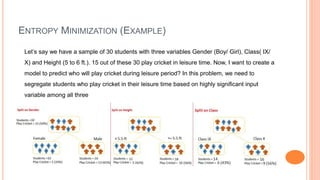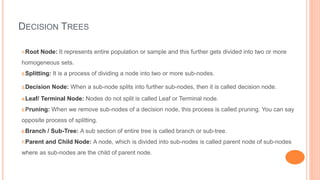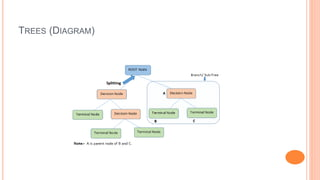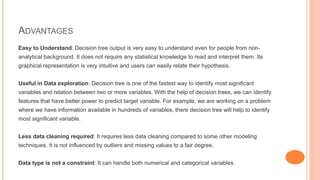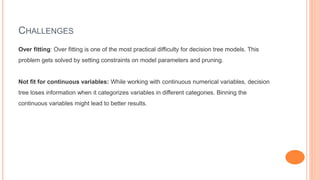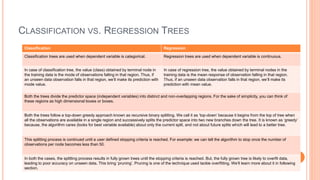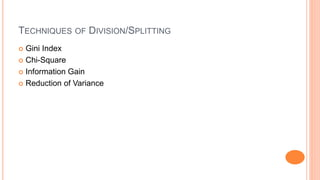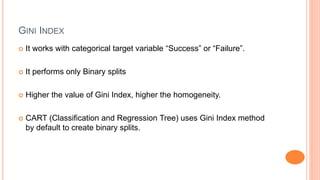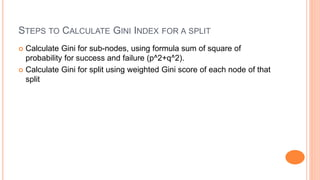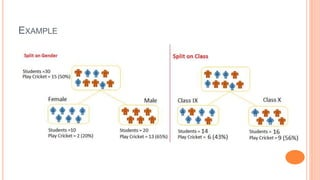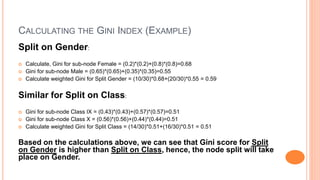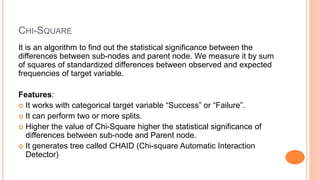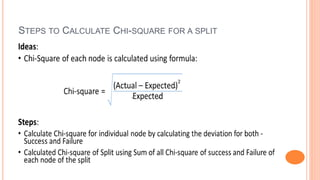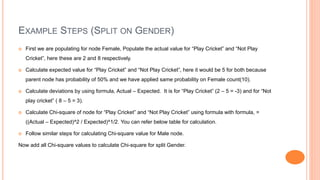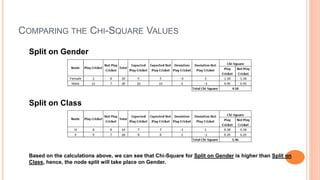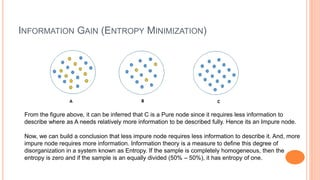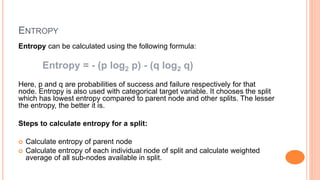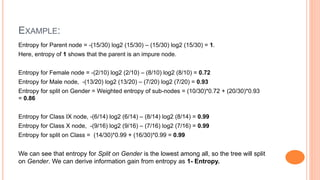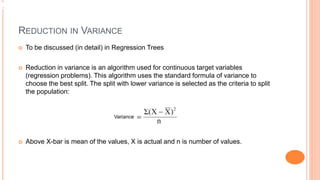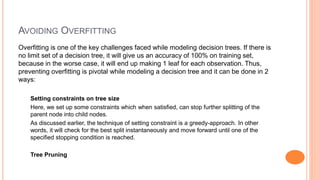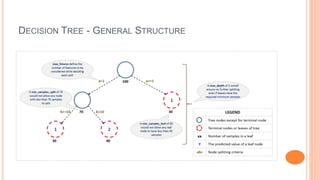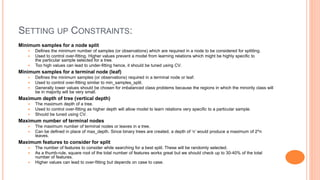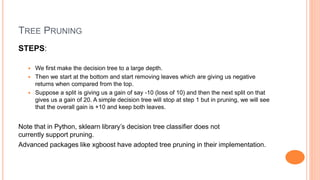1 de 29
Anúncio

### Decision Trees for Classification: A Machine Learning Algorithm

1. DECISION TREES Classification https://palin.co.in/
2. CART CLASSIFICATION AND REGRESSION TREES  Classification Trees  Regression Tress
3. ABOUT:  Very old technique  This technique has become a basis for more sophisticated Classification Techniques such as:  Random Forests  Gradient Boosting  Works on the principal of Entropy Minimization
4. ENTROPY MINIMIZATION  Finding out the greatest differentiator amongst all the input variables so that the population dataset can be divided into sub-populations with minimum entropy.  This means, the sub-populations should be Homogeneous (and Heterogeneous to each other).  The best differentiator is chosen to divide the dataset into different nodes of the parent dataset.  The process is repeated until all sub-populations are homogeneous.
5. ENTROPY MINIMIZATION (EXAMPLE) Let’s say we have a sample of 30 students with three variables Gender (Boy/ Girl), Class( IX/ X) and Height (5 to 6 ft.). 15 out of these 30 play cricket in leisure time. Now, I want to create a model to predict who will play cricket during leisure period? In this problem, we need to segregate students who play cricket in their leisure time based on highly significant input variable among all three
6. DECISION TREES 1.Root Node: It represents entire population or sample and this further gets divided into two or more homogeneous sets. 2.Splitting: It is a process of dividing a node into two or more sub-nodes. 3.Decision Node: When a sub-node splits into further sub-nodes, then it is called decision node. 4.Leaf/ Terminal Node: Nodes do not split is called Leaf or Terminal node. 5.Pruning: When we remove sub-nodes of a decision node, this process is called pruning. You can say opposite process of splitting. 6.Branch / Sub-Tree: A sub section of entire tree is called branch or sub-tree. 7.Parent and Child Node: A node, which is divided into sub-nodes is called parent node of sub-nodes where as sub-nodes are the child of parent node.
7. TREES (DIAGRAM)
8. ADVANTAGES Easy to Understand: Decision tree output is very easy to understand even for people from non- analytical background. It does not require any statistical knowledge to read and interpret them. Its graphical representation is very intuitive and users can easily relate their hypothesis. Useful in Data exploration: Decision tree is one of the fastest way to identify most significant variables and relation between two or more variables. With the help of decision trees, we can identify features that have better power to predict target variable. For example, we are working on a problem where we have information available in hundreds of variables, there decision tree will help to identify most significant variable. Less data cleaning required: It requires less data cleaning compared to some other modeling techniques. It is not influenced by outliers and missing values to a fair degree. Data type is not a constraint: It can handle both numerical and categorical variables.
9. CHALLENGES Over fitting: Over fitting is one of the most practical difficulty for decision tree models. This problem gets solved by setting constraints on model parameters and pruning. Not fit for continuous variables: While working with continuous numerical variables, decision tree loses information when it categorizes variables in different categories. Binning the continuous variables might lead to better results.
10. CLASSIFICATION VS. REGRESSION TREES Classification Regression Classification trees are used when dependent variable is categorical. Regression trees are used when dependent variable is continuous. In case of classification tree, the value (class) obtained by terminal node in the training data is the mode of observations falling in that region. Thus, if an unseen data observation falls in that region, we’ll make its prediction with mode value. In case of regression tree, the value obtained by terminal nodes in the training data is the mean response of observation falling in that region. Thus, if an unseen data observation falls in that region, we’ll make its prediction with mean value. Both the trees divide the predictor space (independent variables) into distinct and non-overlapping regions. For the sake of simplicity, you can think of these regions as high dimensional boxes or boxes. Both the trees follow a top-down greedy approach known as recursive binary splitting. We call it as ‘top-down’ because it begins from the top of tree when all the observations are available in a single region and successively splits the predictor space into two new branches down the tree. It is known as ‘greedy’ because, the algorithm cares (looks for best variable available) about only the current split, and not about future splits which will lead to a better tree. This splitting process is continued until a user defined stopping criteria is reached. For example: we can tell the algorithm to stop once the number of observations per node becomes less than 50. In both the cases, the splitting process results in fully grown trees until the stopping criteria is reached. But, the fully grown tree is likely to overfit data, leading to poor accuracy on unseen data. This bring ‘pruning’. Pruning is one of the technique used tackle overfitting. We’ll learn more about it in following section.
11. TECHNIQUES OF DIVISION/SPLITTING  Gini Index  Chi-Square  Information Gain  Reduction of Variance
12. GINI INDEX  It works with categorical target variable “Success” or “Failure”.  It performs only Binary splits  Higher the value of Gini Index, higher the homogeneity.  CART (Classification and Regression Tree) uses Gini Index method by default to create binary splits.
13. STEPS TO CALCULATE GINI INDEX FOR A SPLIT  Calculate Gini for sub-nodes, using formula sum of square of probability for success and failure (p^2+q^2).  Calculate Gini for split using weighted Gini score of each node of that split
14. EXAMPLE
15. CALCULATING THE GINI INDEX (EXAMPLE) Split on Gender:  Calculate, Gini for sub-node Female = (0.2)*(0.2)+(0.8)*(0.8)=0.68  Gini for sub-node Male = (0.65)*(0.65)+(0.35)*(0.35)=0.55  Calculate weighted Gini for Split Gender = (10/30)*0.68+(20/30)*0.55 = 0.59 Similar for Split on Class:  Gini for sub-node Class IX = (0.43)*(0.43)+(0.57)*(0.57)=0.51  Gini for sub-node Class X = (0.56)*(0.56)+(0.44)*(0.44)=0.51  Calculate weighted Gini for Split Class = (14/30)*0.51+(16/30)*0.51 = 0.51 Based on the calculations above, we can see that Gini score for Split on Gender is higher than Split on Class, hence, the node split will take place on Gender.
16. CHI-SQUARE It is an algorithm to find out the statistical significance between the differences between sub-nodes and parent node. We measure it by sum of squares of standardized differences between observed and expected frequencies of target variable. Features:  It works with categorical target variable “Success” or “Failure”.  It can perform two or more splits.  Higher the value of Chi-Square higher the statistical significance of differences between sub-node and Parent node.  It generates tree called CHAID (Chi-square Automatic Interaction Detector)
17. STEPS TO CALCULATE CHI-SQUARE FOR A SPLIT
18. EXAMPLE STEPS (SPLIT ON GENDER)  First we are populating for node Female, Populate the actual value for “Play Cricket” and “Not Play Cricket”, here these are 2 and 8 respectively.  Calculate expected value for “Play Cricket” and “Not Play Cricket”, here it would be 5 for both because parent node has probability of 50% and we have applied same probability on Female count(10).  Calculate deviations by using formula, Actual – Expected. It is for “Play Cricket” (2 – 5 = -3) and for “Not play cricket” ( 8 – 5 = 3).  Calculate Chi-square of node for “Play Cricket” and “Not Play Cricket” using formula with formula, = ((Actual – Expected)^2 / Expected)^1/2. You can refer below table for calculation.  Follow similar steps for calculating Chi-square value for Male node. Now add all Chi-square values to calculate Chi-square for split Gender.
19. COMPARING THE CHI-SQUARE VALUES Split on Gender Split on Class Based on the calculations above, we can see that Chi-Square for Split on Gender is higher than Split on Class, hence, the node split will take place on Gender.
20. INFORMATION GAIN (ENTROPY MINIMIZATION) From the figure above, it can be inferred that C is a Pure node since it requires less information to describe where as A needs relatively more information to be described fully. Hence its an Impure node. Now, we can build a conclusion that less impure node requires less information to describe it. And, more impure node requires more information. Information theory is a measure to define this degree of disorganization in a system known as Entropy. If the sample is completely homogeneous, then the entropy is zero and if the sample is an equally divided (50% – 50%), it has entropy of one.
21. ENTROPY Entropy can be calculated using the following formula: Entropy = - (p log2 p) - (q log2 q) Here, p and q are probabilities of success and failure respectively for that node. Entropy is also used with categorical target variable. It chooses the split which has lowest entropy compared to parent node and other splits. The lesser the entropy, the better it is. Steps to calculate entropy for a split:  Calculate entropy of parent node  Calculate entropy of each individual node of split and calculate weighted average of all sub-nodes available in split.
22. EXAMPLE: Entropy for Parent node = -(15/30) log2 (15/30) – (15/30) log2 (15/30) = 1. Here, entropy of 1 shows that the parent is an impure node. Entropy for Female node = -(2/10) log2 (2/10) – (8/10) log2 (8/10) = 0.72 Entropy for Male node, -(13/20) log2 (13/20) – (7/20) log2 (7/20) = 0.93 Entropy for split on Gender = Weighted entropy of sub-nodes = (10/30)*0.72 + (20/30)*0.93 = 0.86 Entropy for Class IX node, -(6/14) log2 (6/14) – (8/14) log2 (8/14) = 0.99 Entropy for Class X node, -(9/16) log2 (9/16) – (7/16) log2 (7/16) = 0.99 Entropy for split on Class = (14/30)*0.99 + (16/30)*0.99 = 0.99 We can see that entropy for Split on Gender is the lowest among all, so the tree will split on Gender. We can derive information gain from entropy as 1- Entropy.
23. REDUCTION IN VARIANCE  To be discussed (in detail) in Regression Trees  Reduction in variance is an algorithm used for continuous target variables (regression problems). This algorithm uses the standard formula of variance to choose the best split. The split with lower variance is selected as the criteria to split the population:  Above X-bar is mean of the values, X is actual and n is number of values.
24. AVOIDING OVERFITTING Overfitting is one of the key challenges faced while modeling decision trees. If there is no limit set of a decision tree, it will give us an accuracy of 100% on training set, because in the worse case, it will end up making 1 leaf for each observation. Thus, preventing overfitting is pivotal while modeling a decision tree and it can be done in 2 ways: Setting constraints on tree size Here, we set up some constraints which when satisfied, can stop further splitting of the parent node into child nodes. As discussed earlier, the technique of setting constraint is a greedy-approach. In other words, it will check for the best split instantaneously and move forward until one of the specified stopping condition is reached. Tree Pruning
25. DECISION TREE - GENERAL STRUCTURE
26. SETTING UP CONSTRAINTS: Minimum samples for a node split  Defines the minimum number of samples (or observations) which are required in a node to be considered for splitting.  Used to control over-fitting. Higher values prevent a model from learning relations which might be highly specific to the particular sample selected for a tree.  Too high values can lead to under-fitting hence, it should be tuned using CV. Minimum samples for a terminal node (leaf)  Defines the minimum samples (or observations) required in a terminal node or leaf.  Used to control over-fitting similar to min_samples_split.  Generally lower values should be chosen for imbalanced class problems because the regions in which the minority class will be in majority will be very small. Maximum depth of tree (vertical depth)  The maximum depth of a tree.  Used to control over-fitting as higher depth will allow model to learn relations very specific to a particular sample.  Should be tuned using CV. Maximum number of terminal nodes  The maximum number of terminal nodes or leaves in a tree.  Can be defined in place of max_depth. Since binary trees are created, a depth of ‘n’ would produce a maximum of 2^n leaves. Maximum features to consider for split  The number of features to consider while searching for a best split. These will be randomly selected.  As a thumb-rule, square root of the total number of features works great but we should check up to 30-40% of the total number of features.  Higher values can lead to over-fitting but depends on case to case.
27. TREE PRUNING STEPS:  We first make the decision tree to a large depth.  Then we start at the bottom and start removing leaves which are giving us negative returns when compared from the top.  Suppose a split is giving us a gain of say -10 (loss of 10) and then the next split on that gives us a gain of 20. A simple decision tree will stop at step 1 but in pruning, we will see that the overall gain is +10 and keep both leaves. Note that in Python, sklearn library’s decision tree classifier does not currently support pruning. Advanced packages like xgboost have adopted tree pruning in their implementation.
Anúncio